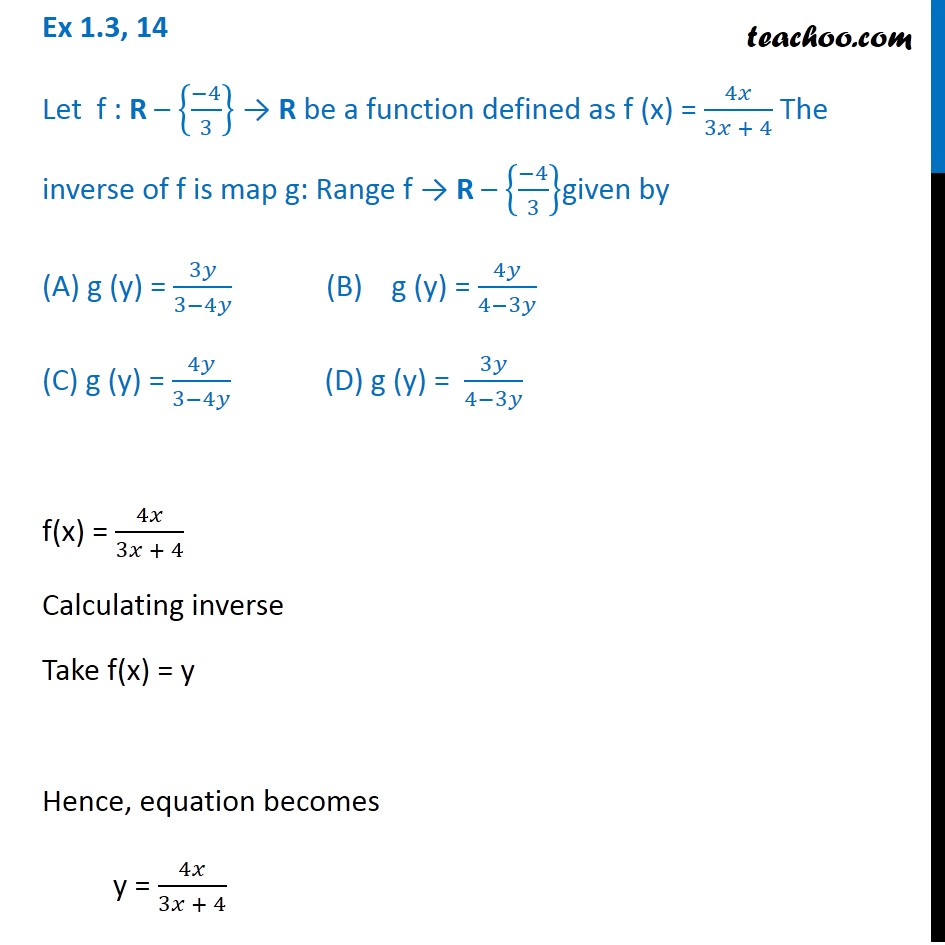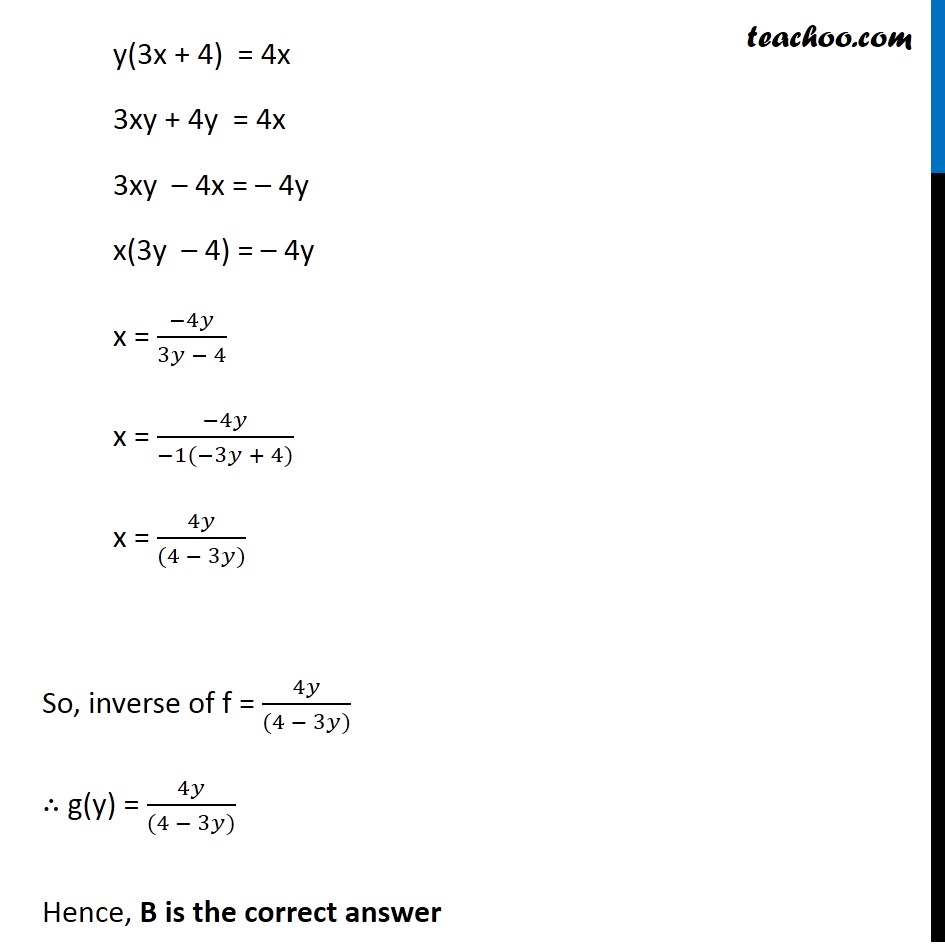Chapter 1 Class 12 Relation and Functions

Class 12
Important Questions for exams Class 12Introducing your new favourite teacher - Teachoo Black, at only ₹83 per month

### Transcript

Ex 1.3, 14 Let f : R – {(−4)/3} → R be a function defined as f (x) = 4𝑥/(3𝑥 + 4) The inverse of f is map g: Range f → R – {(−4)/3}given by (A) g (y) = 3𝑦/(3−4𝑦) (B) g (y) = 4𝑦/(4−3𝑦) (C) g (y) = 4𝑦/(3−4𝑦) (D) g (y) = 3𝑦/(4−3𝑦) f(x) = 4𝑥/(3𝑥 + 4) Calculating inverse Take f(x) = y Hence, equation becomes y = 4𝑥/(3𝑥 + 4) y(3x + 4) = 4x 3xy + 4y = 4x 3xy – 4x = – 4y x(3y – 4) = – 4y x = (−4𝑦)/(3𝑦 − 4) x = (−4𝑦)/(−1(−3𝑦 + 4)) x = 4𝑦/((4 − 3𝑦)) So, inverse of f = 4𝑦/((4 − 3𝑦)) ∴ g(y) = 4𝑦/((4 − 3𝑦)) Hence, B is the correct answer